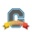# Community

cancel
Showing results for
Did you mean:Community Member

## Students choose which questions to answer on Quiz

How can I let my students pick 5 out of the 6 possible questions in a Quiz on their own, not be given 5 randomly chosen questions by Canvas?

1 Solution

Accepted SolutionsCommunity Coach

@sokolovi , the only way we've been able to accomplish this on our campus is to use a "text no question" type question and put all of the possible questions/options here. Then we have a blank essay question for however many questions we want students to answer. So for example if you wanted students to define 5 of the following 6 terms we'd set up something like this (see below) where the first box is the "text no question" and then the next 5 boxes (only 2 are showing up in this screen shot) are the essay questions where students enter their responses to the five terms/questions they picked.4 RepliesCommunity Coach

@sokolovi , the only way we've been able to accomplish this on our campus is to use a "text no question" type question and put all of the possible questions/options here. Then we have a blank essay question for however many questions we want students to answer. So for example if you wanted students to define 5 of the following 6 terms we'd set up something like this (see below) where the first box is the "text no question" and then the next 5 boxes (only 2 are showing up in this screen shot) are the essay questions where students enter their responses to the five terms/questions they picked.Community Participant

I also want to do this. I often give options to my students:  pick one of two essay questions to answer, pick 8 out of 10 short answer questions, etc.  The method described in the answer above does not satisfy this need.Community Member

Does anyone know how to do this with multiple choice questions?Community Participant

I don't know if you found a solution, but for essay questions, this is a way it ought to work. Let's say I want students to pick 5 out of 10 questions to answer. For simplicity, let's say I want this to be 100 points on the exam. I will set up 10 questions, and I will make each question worth 10 points. My exam is worth 100 points 10x10

I tell the students to answer 5 and that I will assign 20 points per answer. The questions they don't answer receive 0 points. The ones they do answer receive up to 20 points. 5x20 = 100 points possible on the test.

If Canvas balks at giving 20 points for a 10 point question (which it shouldn't), I could use the fudge points feature to add in the points they deserve or assign points to the unanswered questions (though that could get complicated).

This also gets a little more complicated when you have different numbers that they can choose, such as 9 out of 10. Do the math and make sure you can divide the total number of points for that part of the exam by the total number of questions and by the number you want them to answer. For example, for 6 out of 10, then if the total points on the exam (or that part of the exam) were 60 points, you could assign 6 points to each question on the quiz (6x10), but when grading assign 10 points to each of the 6 they answer. Or 30 points, 3 points per question, but give 5 points for each of the 6 questions they answer.

For letting students choose X out of Y, make sure that the total points is divisible by X and by Y, and it should work. 3 out of 4 would work if it was worth 12 points. Each question would be worth 3 points, but when you grade you would give 4 points to the ones they chose to answer.